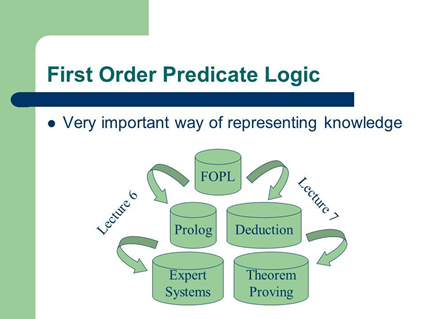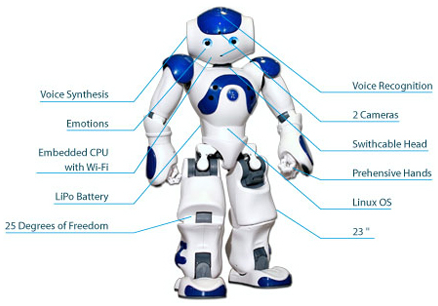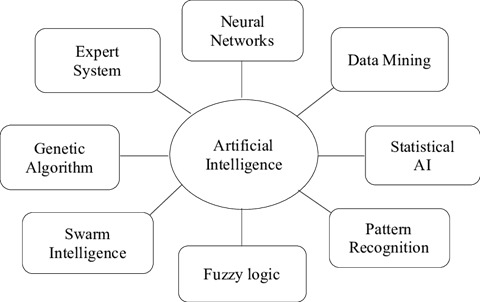### Latest Artificial Intelligence Interview Questions and Answers

Artificial Intelligence has come a long way from being the stuff of science fiction movies and books to becoming an integral part of our daily lives. Today, AI is one of the fastest growing global industries. Investments and experiments in AI have been taking place all around the world. Given its unimaginably wide range of uses; AI is a field of expertise that is set to grow in a very huge way over the coming years. AI professionals are among the highest paid in the field of IT.

1st Round: Artificial Intelligence Basic interview questions and answers:

Q1)Define Artificial Intelligence?

Ans: Artificial Intelligence is a part of computer science that aims to create machine that are intelligent and seek to work and react the way humans do.

Q2)What to you understand by an artificial intelligence Neural Network?

Ans: An Artificial Intelligence Neural Network is a mathematical model that mimes the way the human brain works. What Artificial Intelligence Neural Networks do is that they allow the machine to think and learn in much the same way we do, by giving them the capability to recognize functions such as speech and objects.

Q3)In which areas can AI (Artificial Intelligence) can be used?

Ans: The realm of Artificial Intelligence is very wide and large. This includes Humanoid robot, Speech recognition, Bio-informatics, Computer software, Computing, aeronautics, Space, etc.

Q4)Which is a programming language not commonly used for AI?

Ans: Perl.

Q5)How do you define Prolog in AI?

Ans: IT is a logic-based programming language.

Q6)What are the differences between strong AI and weak AI?

Ans: At a basic level, AI is said to be strong when it claims that computers can be made to think at a level that is almost equal to that of humans. On the other hand, weak AI simply assumes that some features that look like and behave like human intelligence can be fused into a computer system to make it more effective and useful.

Q7)How do you differentiate between statistical AI and Classical AI?

Ans: The statistical branch of AI is one that deals with what is called “inductive” thought or thinking like humans in deducting logic. This could include discerning a set of patterns, forming or building a trend, and so on. Classical AI is more “deductive” or what may be called “declarative” in nature. It uses factors to come to conclusions. C++ is extensively used in statistical AI while for classical AI, it is LISP.

Q8)What are alternate, artificial, compound and natural keys?

Ans: This is how we can define these terms:

Alternate Key: Alternate Keys is excluding primary keys of all candidate keys.

Artificial Key: Artificial key is when no obvious key either stands alone or a compound is available. This leaves it with no option other than to create a key, which it does by assigning a number to each record or occurrence.

Compound Key: Integrating multiple elements to create a unique identifier for the construct becomes necessary when no single data element that uniquely defines the occurrence within a construct is available. This is known as Compound Key.

Natural Key: One of the data elements stored within a construct is a Natural Key. It is utilized as the primary key.

Q9)What is the basis for a production rule?

Ans: A set of rules and a sequence of steps is what the production rule consists of.

Q10) Less memory is consumed by which search method?

Ans: The method that takes less memory is the “depth first search”.

Q11)Which approach is ideal for game playing problem?

Ans: The Heuristic approach is best suited for game playing problem. This is because it uses the technique based on intelligent guesswork. We can think of human-machine chess, which uses hundreds of thousands of positions to form its moves.

Q12)Which search method  is the A* algorithm based on?

Ans: The best first search method is the basis for A* algorithm. This is because this method facilitates quick choice of path. Also, all characteristics lie in A* algorithm.

Q13)What does a hybrid Bayesian network have?

Ans: A hybrid Bayesian network has both discrete and continuous variables.

Q14)What is agent in artificial intelligence?

Ans: An agent is anything that perceives its environment through the use of sensors and works in this environment. Robots, Programs, and Humans are examples of agents.

Q15)What does Partial order or planning entail?

Ans: Partial order planning constructs a plan piece by piece. So, it entails searching over the space of possible plans rather than searching over possible situations it involves.

Q16) Mention two different kinds of steps that we can take in constructing a plan

Ans: a) Add an operator or action

b) Add an ordering constraint between operators

Q17) Which is a property a logical rule-based system that is not considered desirable?

Ans: A logical rule-based system considers attachment an undesirable property.

Q18) What do you understand by a neural Network in Artificial Intelligence?

Ans: The neural network in AI is built just like that of humans: in helping to receive and process the data and give the output that is based on the algorithm and empirical data.

Q19)When is an algorithm considered done?

Ans: An algorithm is considered completed when it ends with a solution when one exists.

Q20)Define a heuristic function

Ans: A heuristic function is one that arranges alternatives in search algorithms at each branching step. This function makes use of the available information to decide which branch to follow.

Q21)What function does the third component of the planning system have?

Ans: The function of the third component in a planning system is to detect a solution to a problem when it is found.

Q22)Define “Generality” in AI

Ans: Generality is the measure or level of ease with which the method can be adapted to various domains of the application.

Q23)How does a top-down parser work?

Ans: A top-down parser assumes a sentence initially and then successively goes about predicting lower level constituents up to a point where the individual pre-terminal symbols are written.

Q24)What is the difference between breadth first search and best first search in artificial intelligence?

Ans: Breadth first search and best first search are two strategies in AI. They share a degree of similarity. In best first search, the nodes are expanded in accordance with the evaluation function, whereas in breadth first search, we express a node is expanded in accordance to the cost function of the parent node.

Q25)What do you understand of frames and scripts in Artificial Intelligence?

Ans: Frames are a variant of semantic networks. Semantic networks constitute one of the popular ways of presenting non-procedural knowledge in an expert system. An artificial data structure-related frame divides knowledge into substructure by representing “stereotyped situations’.

Scripts are more or less the same as frames. The only aspect at which it differs from frames is that the values that fill the slots must be ordered. Scripts are used in systems that understand natural language for the purpose of organizing a knowledge base in a way the system understands.

Q26)Expand FOPL and explain its role in Artificial Intelligence

Ans: FOPL stands for First Order Predicate Logic. This is the role of FOPL in AI:

a) It is a language which expresses assertions

b) It is an inference system with a deductive apparatus, by which we can draw our conclusions from such assertion

c) FOPL is a set theory- based semantic

Q27)   What does the language of FOPL consists of?

FOPL consists of the following:

a) A set of constant symbols

b) A set of variables

c) A set of predicate symbols

d) A set of function symbols

e) The logical connective

f) The Universal Quantifier and Existential Qualifier

g) A special binary relation of equality

Q28) When making an online search for ‘Artificial Intelligence’, mention the search agent which operates by interleaving computation and action

Ans:For online search, AI will first act and then observe the environment.

Q29) Name the search algorithm that use a limited amount of memory in online search?

Ans:RBFE and SMA* are search algorithms that use a limited amount of memory to solve any kind of problem that A* can’t.

Q30) Where is the Bayes rule used in AI?

Ans: Bayes rule can be used in Artificial Intelligence to answer the probabilistic queries conditioned on one piece of evidence.

Q31) How many terms are required for building a Bayes model?

Ans: Three terms are required for building a Bayes model in AI: one conditional probability and two unconditional probabilities.

Q32) What is the consequence between a node and its predecessors while creating Bayesian Network?

Ans: The consequence between a node and its predecessors while creating Bayesian Network is that a node can be conditionally independent of its predecessors.

Q33) How can the Bayesian network be used to answer any query?

Ans: A Bayesian Network can solve any query by summing all the relevant joint entries if it is a representative of the joint distribution.

Q34)What programming fuses inductive methods with the power of first order representations?

Ans: It is inductive logic programming that combines inductive methods with the power of first order representations.

Q35) What needs to be satisfied in Inductive Logic Programming?

Ans: Coming up with a set of sentences for the hypothesis such that the entailment constraint is satisfied is the objective of an Inductive Logic Programming.

Q36)How many literals are available in top-down inductive learning methods and which are they?

Ans: The following three literals are available in top-down inductive learning methods:

a) Predicates

b) Equality and Inequality

c) Arithmetic Literals

Q37) Which algorithm is used to invert a complete resolution strategy?

Ans: Inverse Resolution, in being a complete algorithm for learning first order theories, inverts a complete resolution.

Q38) What kind of signal is used in speech recognition?

Ans: Acoustic signal is used to identify a sequence of words in speech recognition.

Q39) Which model gives the probability of each word following each word in speech recognition?

Ans: In AI, the probability of each word following each other word in speech recognition is offered by the Biagram model.

Q40) Name the algorithm that is used to solve temporal probabilistic reasoning

Ans: HMM (Hidden Markov Model) is used, independent of transition and sensor model, for this purpose.

Q41) What is Hidden Markov Model (HMMs) used for?

Ans: Hidden Markov Models re used in almost all current speech recognition systems since thy are a ubiquitous tool for modelling time series data or for modeling sequence behavior.

Q42) In Hidden Markov Model, how does one describe the state of the process?

Ans: It is through a ‘Single Discrete Random Variable’.

Q43) What are the possible values of the variable in HMM’s?

Ans: The possible values of the variable in HMM’s is the ‘Possible States of the World’.

Q44) Where is the additional variable added in HMM?

Ans: The additional variable added in HMM is done by adding the additional state variables to a temporal model while staying within the HMM network.

Q45) What are semantic analyses used for in AI?

Ans: Semantic analyses are used In Artificial Intelligence for extracting the meaning from the group of sentences semantic analysis.

Q46)What is compositional semantics?

Ans: It is the method by which the meaning of P*Q is determined from P,Q and*.

Q47) How do you solve logical inference in Propositional Logic?

Ans: This can be done by using these:

a)     Logical Equivalence

b)     Validity

c)      Satisfying ability

Q48)Which is the process in which different logical expression look identical?

Ans: The ‘Unification’ process makes different logical expressions look identical. Lifted inferences need to find substitutes that can make different expressions look identical. This is the meaning of the process called unification.

Q49) Which algorithm takes two sentences and returns a unifier in ‘Unification and Lifting’?

Ans: The algorithm that takes two sentences and returns a unifier in ‘Unification and Lifting’ is ‘Unify’ algorithm.

Q50)Name the most straight forward approach for planning algorithm

Ans: It is State space search. The reason is this: it takes account everything for finding a solution.

2nd Round: Artificial Intelligence Technical Interview Questions and answers:

Q1). What is FOPL?

Ans: FOPL stands for First-order Predicate logic. It is a collection of formal systems. Its constituents are described in the two parts of its name: the predicate and a subject. In FOPL, the predicate holds the potential for defining or modifying the subject’s properties.Q2). What are the components of Robotics?

Ans: The following parts are required to construct a robot:

1.  power Supply: Can be any power source such as batteries, solar power, etc. Actuators: Needed to convert energy into movement
2. Electric motors (AC/DC): For rotational movement
3. Pneumatic Air Muscles: Used for contracting when air gets sucked into them
4. Muscle Wires: These are used to pass electric current during contraction
5. Ultrasonic and Piezo Motors: These parts are used in industrial robots
6. Sensors: Generic sensors, with which the surroundings are measured.Q3). How do you describe the core differences between supervised, unsupervised and reinforcement learning?

Ans:

Types——>

Supervised Learning

Unsupervised Learning

Reinforcement Learning

Definition

1. Training set consists of both predictors and predictions
2. Training set comes with only predictors in the data set.
3. This can be used to carry out state of art results on any task

Algorithm

1. Linear and logistic regression, support vector machine, native Bayes
2. K-means, clustering algorithm, dimensionality reduction algorithm
3. Q-learning, State-action-reward-state-action (SARSA), Deep Q Network (DQN)

Uses

1. Image recognition, Speech, Recognition, Forecasting
2. Pre-Process the data, Pre-train Supervised Learning algorithm.
3. Warehouses, Inventory Management, Delivery Management, Power system financial, system

Q4). What are the branches of AI?

Ans:1. Neural networks: In AI, an artificial neuron network (ANN) is an arithmetic model that is based on the foundation and functionality of various biological neural networks.
2. Data mining: Artificial intelligence uses data mining techniques for solving issues related to diagnosis, segmentation, classification and predictions.
3. Statistical AI: Statistical AI deals with domain models in which both uncertainty and complexity are displayed in rational structures.
4. Pattern recognition: This property of AI runs on the apprehension of regularities and patterns in data.
5. Fuzzy logic: Fuzzy logic is a type of multi-valued logic where the truth values of the variables may vary anywhere between 0 and 1.
6. Swarm Intelligence: This is the term for an AI model that is inspired by biology, on the behavior of common swarm forming animals such as bees, ants etc.
7. Genetic algorithm: The genetic algorithm is a systematic combination of adaptive self-learning algorithm that derives from the concept of genetics.
8. Expert system: The expert system is implemented in designing systems to give them the capability of miming the human decision-making ability.

HR Round:

What is your experience in having worked in projects involving AI?

Explain your problem-solving abilities in AI with demonstrated examples

What future do you see for yourself in this organization as an AI specialist?

Do you believe you have the capability for taking the organization forward with your AI skillset and knowledge?

How far do you see AI taking mankind over the next fifty years? Do you think you have a role to play in it?

Conclusion:

AI has been around for a few decades now. But the field has been given a new lease of life following a few developments. These include the core aspects of AI: there has been a change in the power of computing from the time AI came into existence; the availability of data, central to AI, has grown multifold, and algorithms have improved by leaps and bounds.

Artificial Intelligence is today considered one of the technologies that have the potential to change most aspects of human life. Whether it will be for good or otherwise largely rests in our hands. There is a worldwide movement towards adaption of AI for everything from agriculture to aviation. This makes AI the field to be in for the next generation.